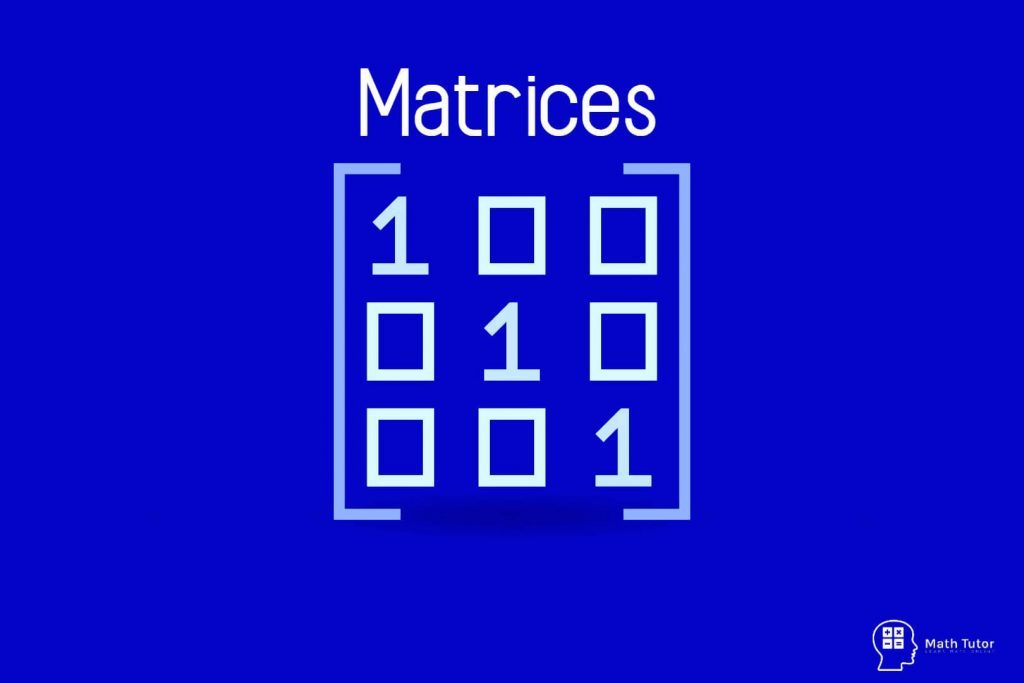## Matrices

Matrices are, rectangular block of numbers arranged in to rows and columns. For example, There are some unique terms that we should know when we are dealing with matrices. Dimensions of Matrix When we consider the above example it has two rows and three columns. So, the dimensions of matrix A is 2 x 3 …